# Management Science Chapter 1 Copyright 2010 Pearson Education

• Slides: 28Management Science Chapter 1 Copyright © 2010 Pearson Education, Inc. Publishing as Prentice Hall 1 -1Chapter Topics n The Management Science Approach to Problem Solving n Model Building: Break-Even Analysis n Computer Solution n Management Science Modeling Techniques n Business Usage of Management Science Techniques n Management Science Models in Decision Support Systems Copyright © 2010 Pearson Education, Inc. Publishing as Prentice Hall 1 -2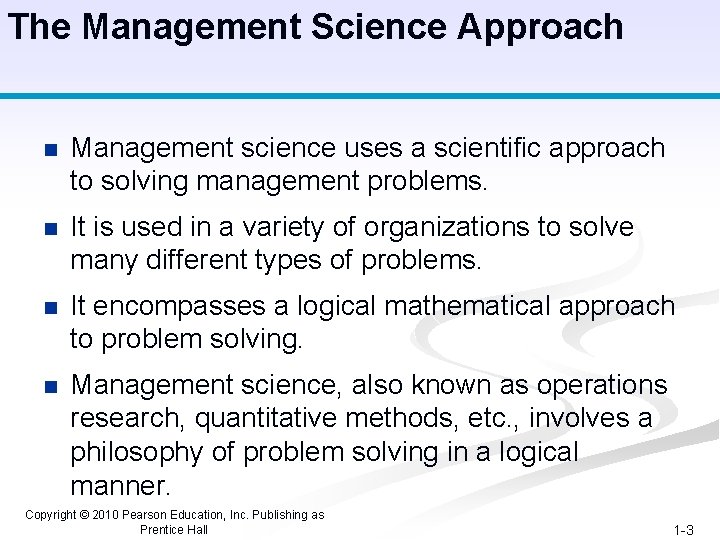The Management Science Approach n Management science uses a scientific approach to solving management problems. n It is used in a variety of organizations to solve many different types of problems. n It encompasses a logical mathematical approach to problem solving. n Management science, also known as operations research, quantitative methods, etc. , involves a philosophy of problem solving in a logical manner. Copyright © 2010 Pearson Education, Inc. Publishing as Prentice Hall 1 -3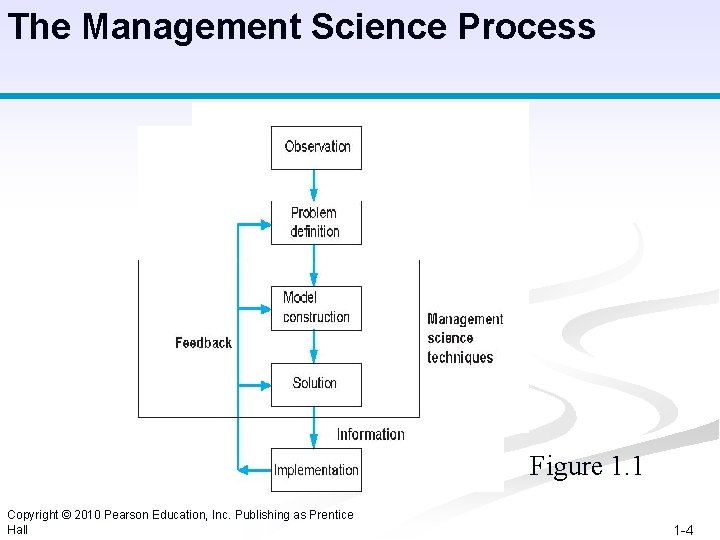The Management Science Process Figure 1. 1 Copyright © 2010 Pearson Education, Inc. Publishing as Prentice Hall 1 -4Steps in the Management Science Process § Observation - Identification of a problem that exists (or may occur soon) in a system or organization. § Definition of the Problem - problem must be clearly and consistently defined, showing its boundaries and interactions with the objectives of the organization. § Model Construction - Development of the functional mathematical relationships that describe the decision variables, objective function and constraints of the problem. § Model Solution - Models solved using management science techniques. § Model Implementation - Actual use of the model or its solution. Copyright © 2010 Pearson Education, Inc. Publishing as Prentice Hall 1 -5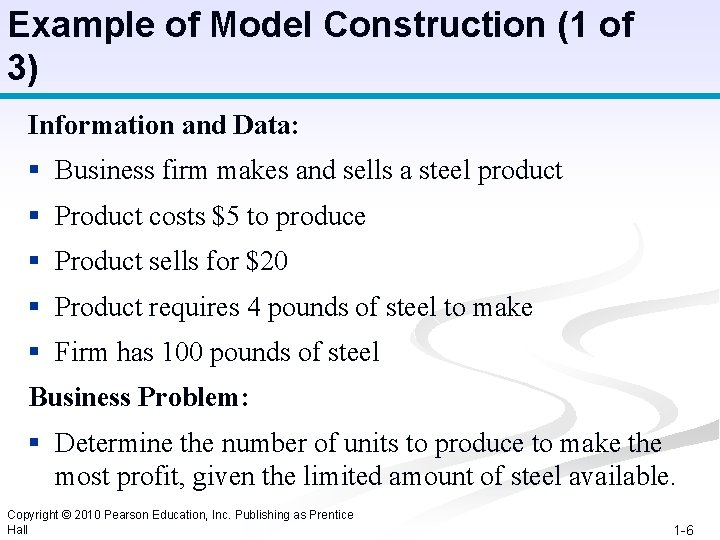Example of Model Construction (1 of 3) Information and Data: § Business firm makes and sells a steel product § Product costs \$5 to produce § Product sells for \$20 § Product requires 4 pounds of steel to make § Firm has 100 pounds of steel Business Problem: § Determine the number of units to produce to make the most profit, given the limited amount of steel available. Copyright © 2010 Pearson Education, Inc. Publishing as Prentice Hall 1 -6Example of Model Construction (2 of 3) Variables: X = # units to produce (decision variable) Z = total profit (in \$) Model: Z = \$20 X - \$5 X (objective function) 4 X = 100 lb of steel (resource constraint) Parameters: values) \$20, \$5, 4 lbs, 100 lbs (known Formal Specification of Model: maximize Z = \$20 X - \$5 X subject to 4 X = 100 Copyright © 2010 Pearson Education, Inc. Publishing as Prentice Hall 1 -7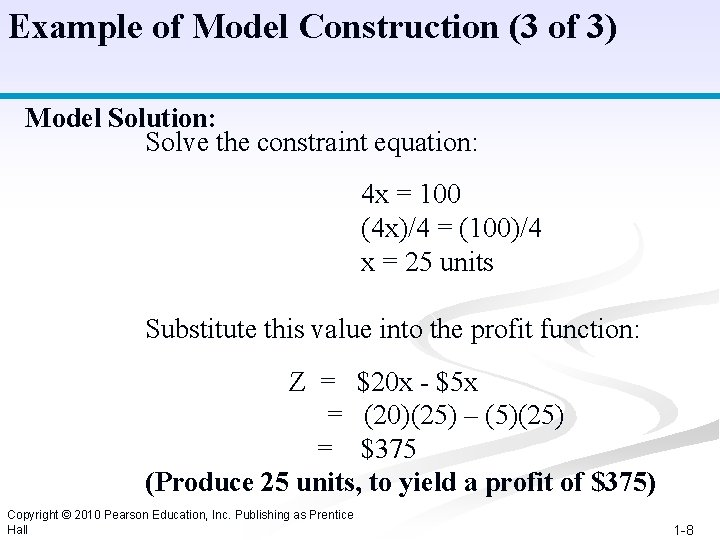Example of Model Construction (3 of 3) Model Solution: Solve the constraint equation: 4 x = 100 (4 x)/4 = (100)/4 x = 25 units Substitute this value into the profit function: Z = \$20 x - \$5 x = (20)(25) – (5)(25) = \$375 (Produce 25 units, to yield a profit of \$375) Copyright © 2010 Pearson Education, Inc. Publishing as Prentice Hall 1 -8Model Building: Break-Even Analysis ■ Used to determine the number of units of a product to sell or produce that will equate total revenue with total cost. ■ The volume (number of products produced) at which total revenue equals total cost is called the break-even point. ■ Profit at break-even point is zero. Copyright © 2010 Pearson Education, Inc. Publishing as Prentice Hall 1 -9Model Building: Break-Even Analysis Model Components n Fixed Cost (cf) - costs that remain constant regardless of number of units produced. (e. g. Rent, taxes, management salaries, insurance, heating etc. ) n Variable Cost (cv) - unit production cost of product. (including raw material, labor, resources, packaging, material handling, transportation) n Volume (v) – the number of units produced or sold n Total variable cost (vcv) - function of volume (v) and unit variable cost. Copyright © 2010 Pearson Education, Inc. Publishing as Prentice Hall 1 -10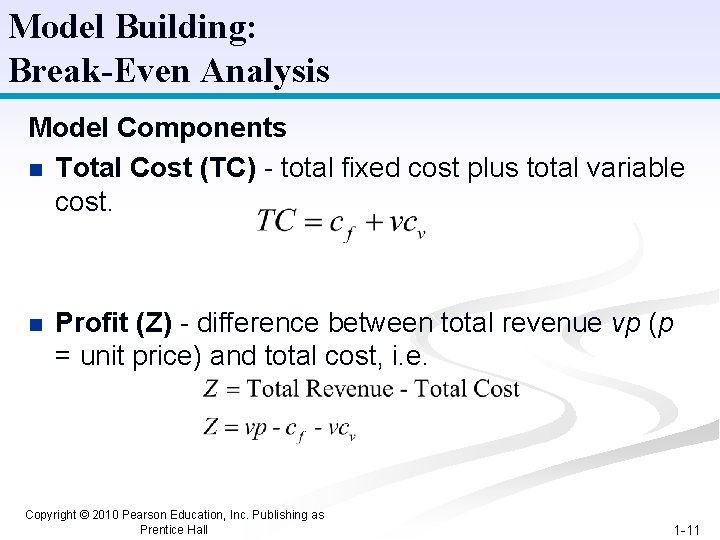Model Building: Break-Even Analysis Model Components n Total Cost (TC) - total fixed cost plus total variable cost. n Profit (Z) - difference between total revenue vp (p = unit price) and total cost, i. e. Copyright © 2010 Pearson Education, Inc. Publishing as Prentice Hall 1 -11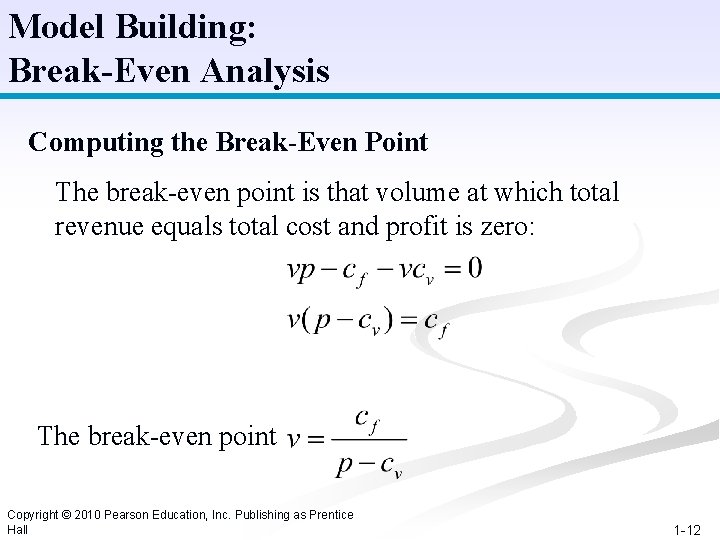Model Building: Break-Even Analysis Computing the Break-Even Point The break-even point is that volume at which total revenue equals total cost and profit is zero: The break-even point Copyright © 2010 Pearson Education, Inc. Publishing as Prentice Hall 1 -12Model Building: Break-Even Analysis Example: Western Clothing Company Fixed Costs: cf = \$10000 Variable Costs: cv = \$8 per pair Price : p = \$23 per pair The Break-Even Point is: v = (10, 000)/(23 -8) = 666. 7 pairs Copyright © 2010 Pearson Education, Inc. Publishing as Prentice Hall 1 -13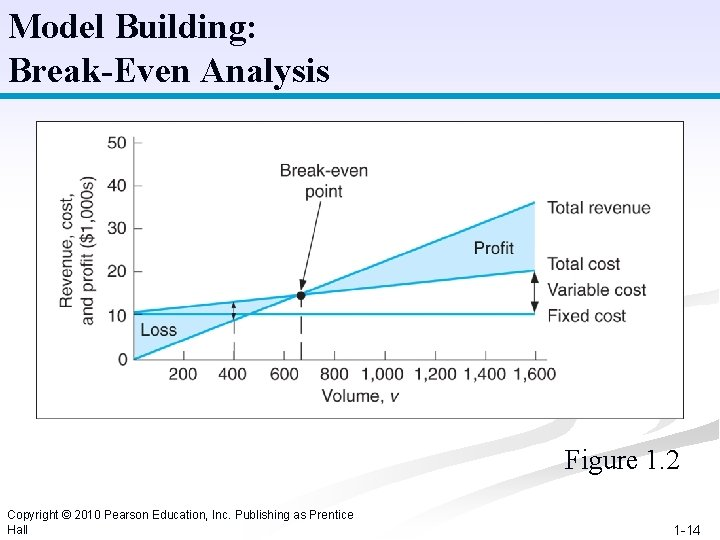Model Building: Break-Even Analysis Figure 1. 2 Copyright © 2010 Pearson Education, Inc. Publishing as Prentice Hall 1 -14Model Building: Break-Even Analysis Example: Western Clothing Company Fixed Costs: cf = \$10000 Variable Costs: cv = \$8 per pair Price : p = \$30 per pair The Break-Even Point is: v = (10, 000)/(30 -8) = 454. 5 pairs Copyright © 2010 Pearson Education, Inc. Publishing as Prentice Hall 1 -15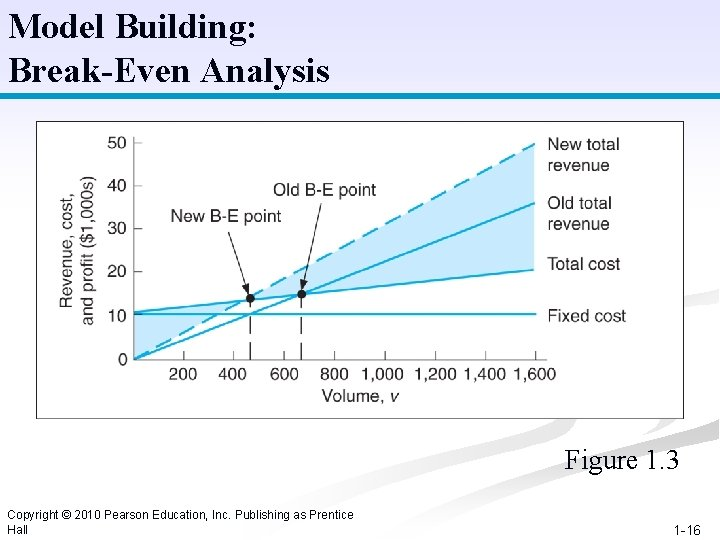Model Building: Break-Even Analysis Figure 1. 3 Copyright © 2010 Pearson Education, Inc. Publishing as Prentice Hall 1 -16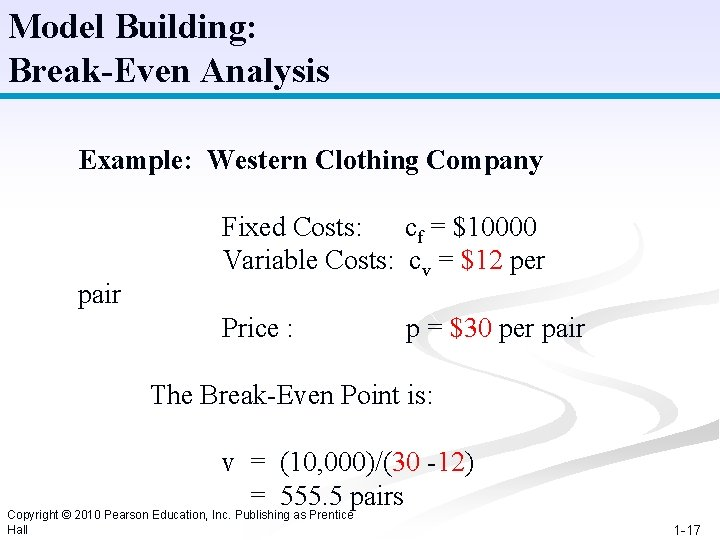Model Building: Break-Even Analysis Example: Western Clothing Company pair Fixed Costs: cf = \$10000 Variable Costs: cv = \$12 per Price : p = \$30 per pair The Break-Even Point is: v = (10, 000)/(30 -12) = 555. 5 pairs Copyright © 2010 Pearson Education, Inc. Publishing as Prentice Hall 1 -17Model Building: Break-Even Analysis Figure 1. 4 Copyright © 2010 Pearson Education, Inc. Publishing as Prentice Hall 1 -18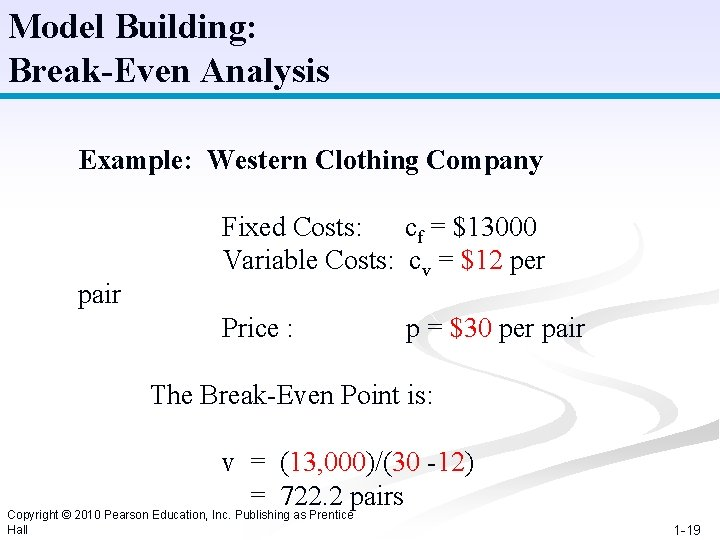Model Building: Break-Even Analysis Example: Western Clothing Company pair Fixed Costs: cf = \$13000 Variable Costs: cv = \$12 per Price : p = \$30 per pair The Break-Even Point is: v = (13, 000)/(30 -12) = 722. 2 pairs Copyright © 2010 Pearson Education, Inc. Publishing as Prentice Hall 1 -19Model Building: Break-Even Analysis Figure 1. 5 Copyright © 2010 Pearson Education, Inc. Publishing as Prentice Hall 1 -20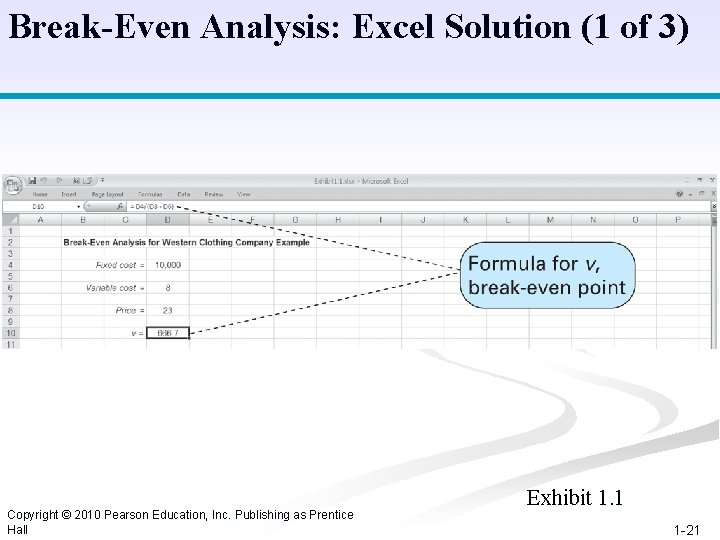Break-Even Analysis: Excel Solution (1 of 3) Copyright © 2010 Pearson Education, Inc. Publishing as Prentice Hall Exhibit 1. 1 1 -21Break-Even Analysis: QM Solution (2 of 3) Exhibit 1. 4 Copyright © 2010 Pearson Education, Inc. Publishing as Prentice Hall 1 -22Break-Even Analysis: QM Solution (3 of 3) Copyright © 2010 Pearson Education, Inc. Publishing as Prentice Hall Exhibit 1. 5 1 -23Classification of Management Science Techniques Figure 1. 6 Modeling Techniques Copyright © 2010 Pearson Education, Inc. Publishing as Prentice Hall 1 -24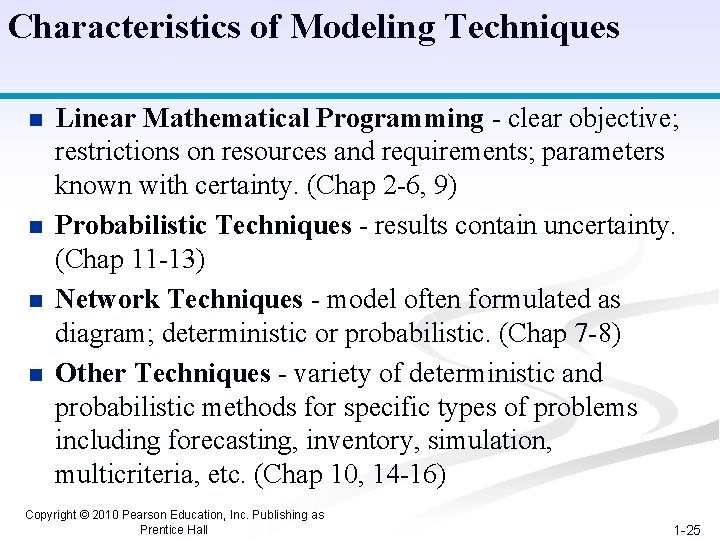Characteristics of Modeling Techniques n n Linear Mathematical Programming - clear objective; restrictions on resources and requirements; parameters known with certainty. (Chap 2 -6, 9) Probabilistic Techniques - results contain uncertainty. (Chap 11 -13) Network Techniques - model often formulated as diagram; deterministic or probabilistic. (Chap 7 -8) Other Techniques - variety of deterministic and probabilistic methods for specific types of problems including forecasting, inventory, simulation, multicriteria, etc. (Chap 10, 14 -16) Copyright © 2010 Pearson Education, Inc. Publishing as Prentice Hall 1 -25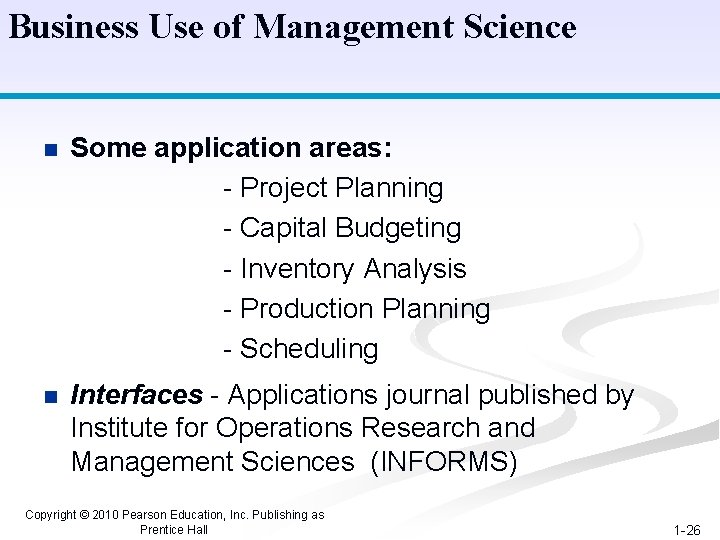Business Use of Management Science n Some application areas: - Project Planning - Capital Budgeting - Inventory Analysis - Production Planning - Scheduling n Interfaces - Applications journal published by Institute for Operations Research and Management Sciences (INFORMS) Copyright © 2010 Pearson Education, Inc. Publishing as Prentice Hall 1 -26Decision Support Systems (DSS) A decision support system is a computer-based system that helps decision makers address complex problems that cut across different parts of an organization and operations. Features of Decision Support Systems n Interactive n Use databases & management science models n Address “what if” questions n Perform sensitivity analysis Examples include: ERP – Enterprise Resource Planning OLAP – Online Analytical Processing Copyright © 2010 Pearson Education, Inc. Publishing as Prentice Hall 1 -27Management Science Models Decision Support Systems (2 of 2) Figure 1. 7 A Decision Support System Copyright © 2010 Pearson Education, Inc. Publishing as Prentice Hall 1 -28